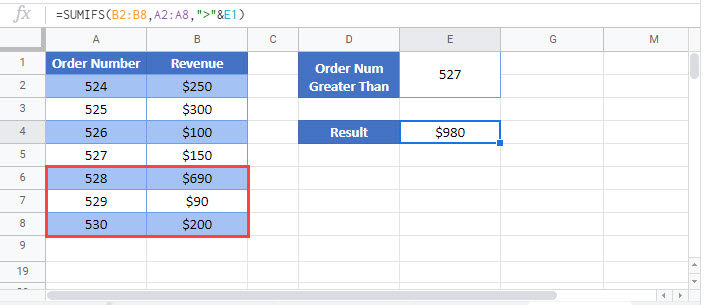# Sum If Greater Than or Equal To – Excel & Google Sheets

This tutorial will demonstrate how to use the SUMIFS Function to sum rows with data greater than (or equal to) a specific value in Excel and Google Sheets.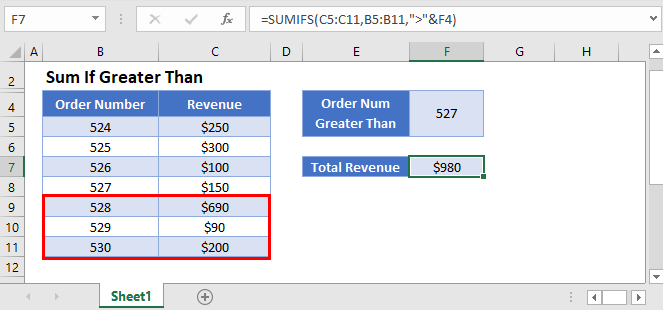## Sum if Greater Than 0

The SUMIFS Function sums data rows that meet certain criteria. Its syntax is: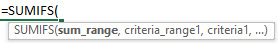This example will sum all Scores that are greater than zero.

``=SUMIFS(C3:C9,C3:C9,">0")``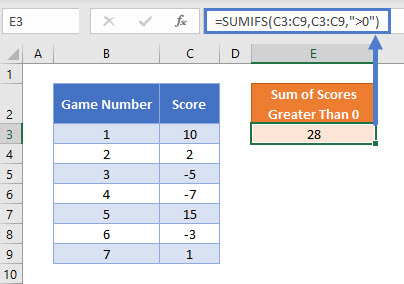Note: The criteria “>0” sums all Scores greater than zero.

## Sum if Greater Than

This example will sum the Revenue for all Order Numbers greater than 527 (Criteria is “>527”).

``=SUMIFS(C3:C9,B3:B9,">527")``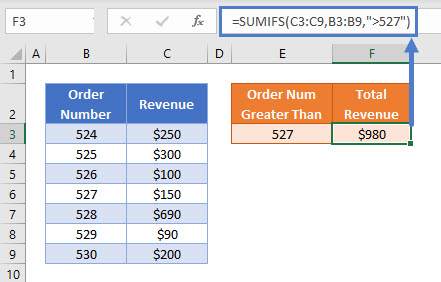### Sum if Greater Than or Equal to

To include Revenue from Order Numbers which are greater than or equal to 527, we can modify the the criteria like so (“>=527”):

``=SUMIFS(C3:C9,B3:B9,">=527")``

### Sum if Greater Than – Cell References

Usually, it is bad practice to “hard-code” values into formulas. Instead, it is more flexible to use a separate cell to define the criteria’s value.

``=SUMIFS(C3:C9,B3:B9,">"&E3)``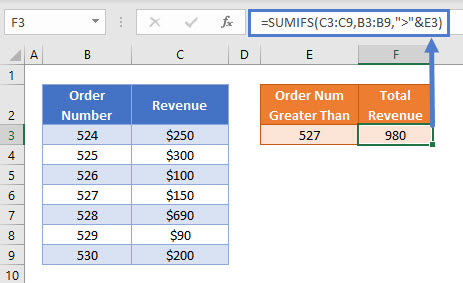Now we add the logical operator within double quotes (“”) and use & to join the operator and the value:

``">"&E3``

Other logical operators can be used in this formula, such as:

• Equal to (“=”&E3)
• Greater than or equal to (“>=”&E3)
• Less than (“<“&E3)
• Less than or equal to (“<=”&E3)
• Not equal to (“<>”&E3)

## Locking Cell References

To make our formulas easier to read, we’ve shown the formulas without locked cell references:

``=SUMIFS(C3:C9,B3:B9,">"&E3)``

But these formulas will not work properly when copy and pasted elsewhere in your file. Instead, you should use locked cell references like this:

``=SUMIFS(\$C\$3:\$C\$9,\$B\$3:\$B\$9,">"&E3)``